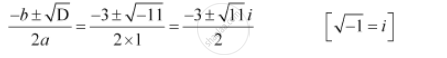CBSE (Arts) Class 11CBSE
Share

# Solve the Quadratic Equation X2 + 3x + 5 = 0 - CBSE (Arts) Class 11 - Mathematics

#### Question

Solve the equation x2 + 3x + 5 = 0

#### Solution

The given quadratic equation is x2 + 3x + 5 = 0

On comparing the given equation with ax2 + bx + c = 0, we obtain

a = 1, b = 3, and c = 5

Therefore, the discriminant of the given equation is

D = b2 – 4ac = 32 – 4 × 1 × 5 =9 – 20 = –11

Therefore, the required solutions areIs there an error in this question or solution?

#### Video TutorialsVIEW ALL 

Solution Solve the Quadratic Equation X2 + 3x + 5 = 0 Concept: Quadratic Equations.
S